NCERT Maths Solutions for Ex 2.1 class 10 Polynomials is the perfect guide to boost up your preparation during CBSE 10th Class Maths Examination.

## RS Aggarwal Class 10 Solutions Chapter 2 Polynomials Ex 2A

These Solutions are part of RS Aggarwal Solutions Class 10. Here we have given RS Aggarwal Class 10 Solutions Chapter 2 Polynomials Ex 2A

Other Exercises

Find the zeros of the following quadratic polynomials and verify the relationship between the zeros and the coefficients:
Question 1.
Solution: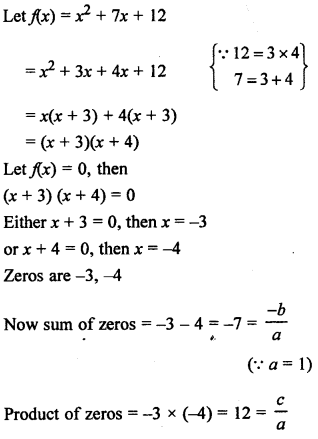Question 2.
Solution:
x² – 2x – 8
Let f(x) = x² – 2x – 8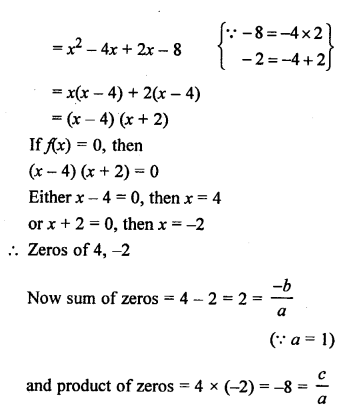Question 3.
Solution: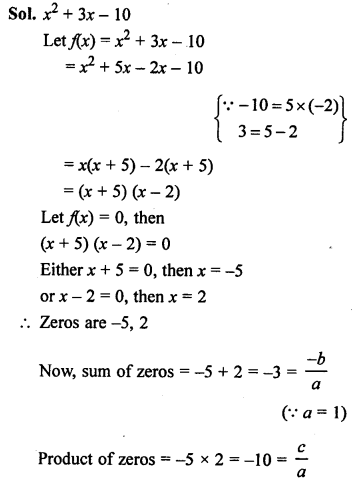Question 4.
Solution:
4x² – 4x – 3Question 5.
Solution:Question 6.
Solution: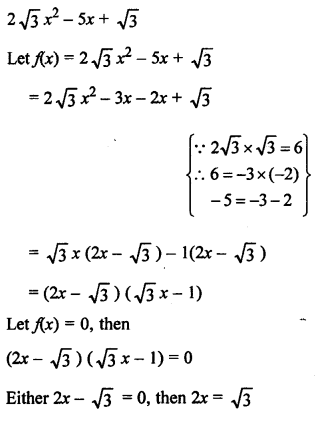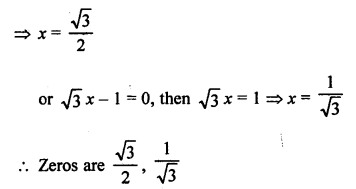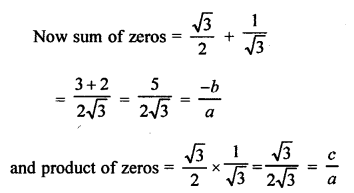Question 7.
Solution: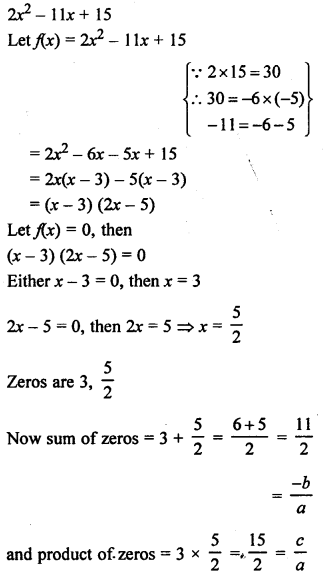Question 8.
Solution:Question 9.
Solution:Question 10.
Solution:Question 11.
Solution: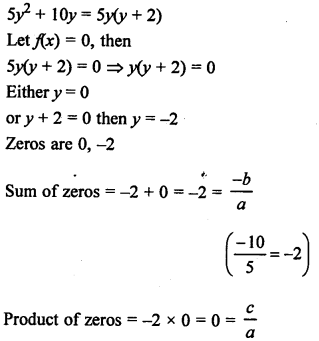Question 12.
Solution: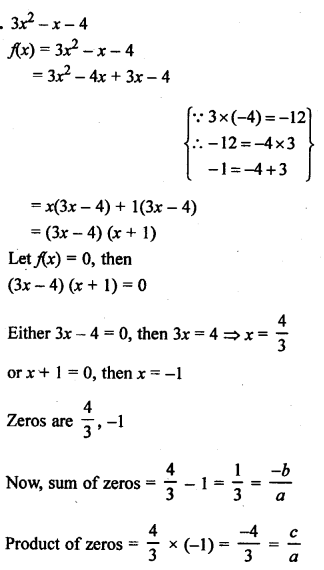Question 13.
Solution:
Zeros of a quadratic polynomial are 2, -6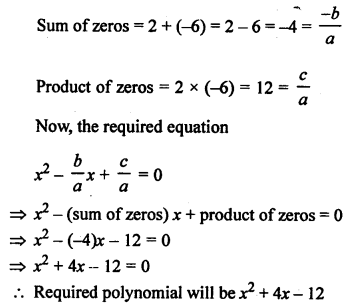Question 14.
Solution: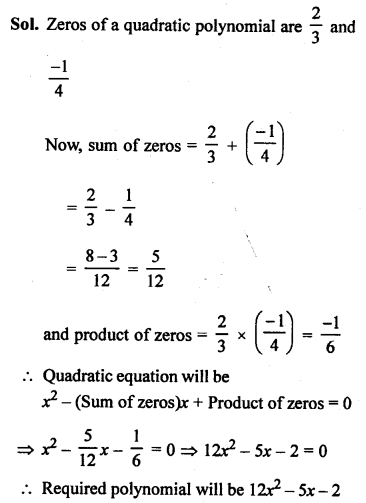Question 15.
Solution:
Sum of zeros = 8
Product of zeros = 12
Quadratic equation will be x² – (Sum of zeros) x + Product of zeros = 0
=> x² – 8x + 12 = 0
=> x² – 6x – 2x + 12 = 0
=> x (x – 6) – 2 (x – 6) = 0
=> (x – 6) (x – 2) = 0
Either x – 6 = 0, then x = 6
or x – 2 = 0, then x = 2
Zeros are 6, 2
and quadratic polynomial is x² – 8x + 12

Question 16.
Solution:
Sum of zeros = 0
and product of zeros = -1
x² – (Sum of zeros) x + Product of zeros = 0
=> x² – 0x – 1 = 0
=> x² – 1= 0
(x + 1)(x – 1) = 0
Either x + 1 = 0, then x = -1 or x – 1 =0, then x = 1
Zeros are 1, -1
and quadratic polynomial is x² – 1

Question 17.
Solution:
Sum of zeros = $$\frac { 5 }{ 2 }$$
Product of zeros = 1
x² – (Sum of zeros) x + Product of zeros = 0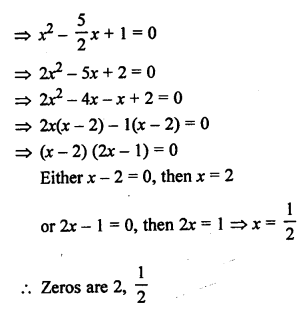and quadratic polynomial is 2x² – 5x + 2

Question 18.
Solution: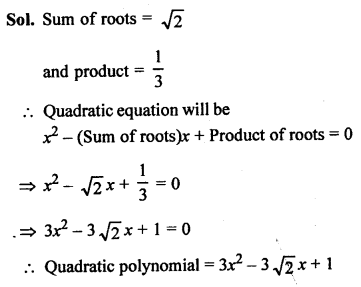Question 19.
Solution:Question 20.
Solution:Question 21.
Solution:
One zero of the given polynomial is $$\frac { 2 }{ 3 }$$=> (x + 3) (x + 3) = 0
x = -3, -3
Hence, other zeros are -3, -3

Hope given RS Aggarwal Solutions Class 10 Chapter 2 Polynomials Ex 2A are helpful to complete your math homework.

If you have any doubts, please comment below. Learn Insta try to provide online math tutoring for you.